##### How to Get Table Size in PostgreSQL

PostgreSQL offers several built-in functions to get the size of databases, tablespaces, tables, etc. If we talk about the table’s size, it can be calculated using the inbuilt pg_relation_size() function. In addition to this, PostgreSQL offers another handy built-in function named pg_size_pretty() that retrieves the table size in an easily understandable format.

This blog post will demonstrate a couple of functions to calculate the table’s size using practical examples. So, let’s get started!

How to Get Table Size in Postgres?

The below syntax is exercised in Postgres to calculate the size of a particular table:

`pg_relation_size(tab_name);`

Here, tab_name is the targeted table whose size needs to be calculated.

Example: How to Find the Table’s Size in Postgres Using pg_relation_size()?

This particular example is going to elaborate the basic concept of the pg_relation_size() function via stepwise instructions:

Step 1: Make a Connection With Database

Execute the “\l” command from SQL Shell to get the list of all the databases:

`\l;`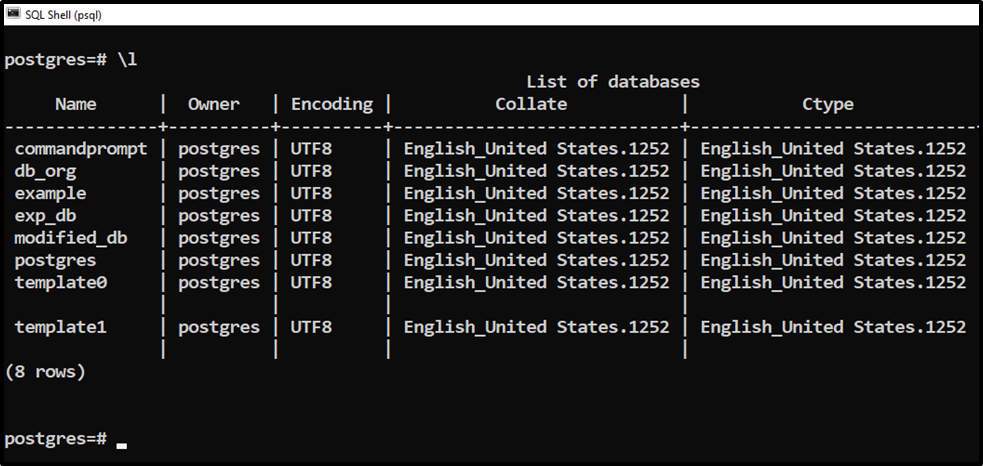Let’s make a connection with a specific database from the available list:

`\c example;`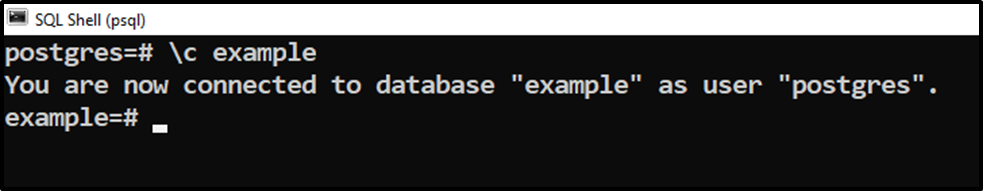We are successfully connected to the “example” database.

Step 2: Select a Table

Once you are connected to a database of your choice, run the “\dt” command to describe the list of relations:

`\dt;`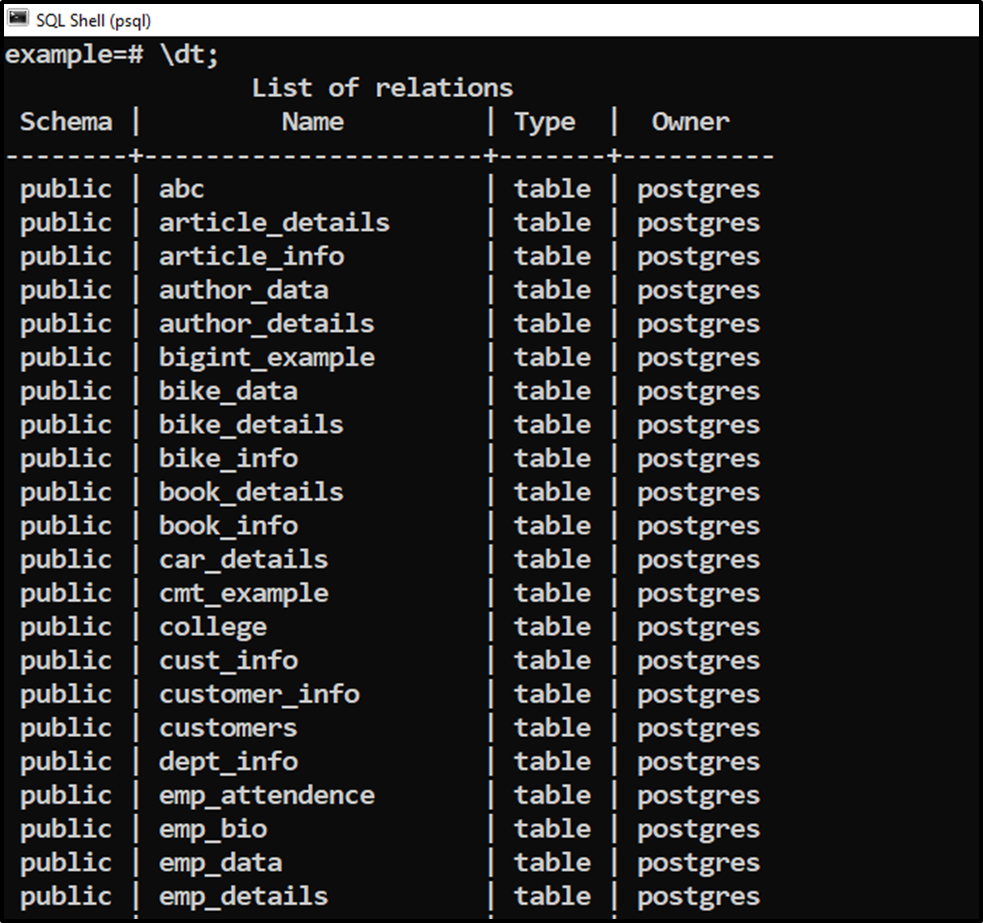Suppose we need to calculate the size of the “article_details” table.

Step 3: Get Table Size

To get the size of the selected table, you must use the “pg_relation_size()” function as follows:

`SELECT pg_relation_size('article_details');`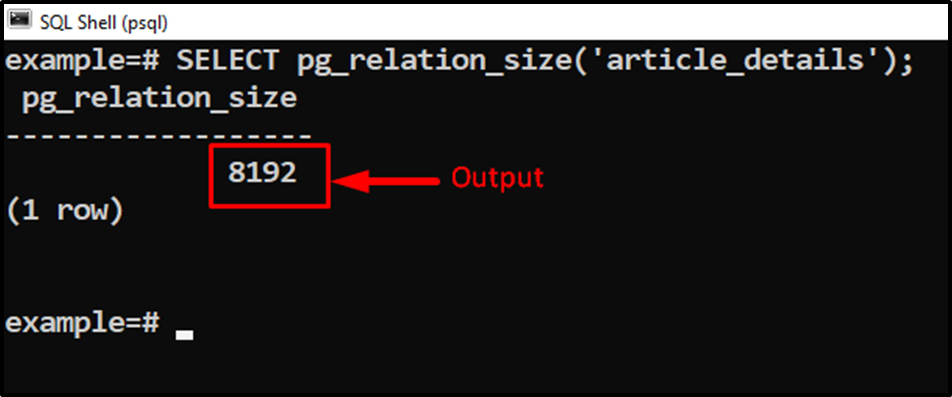The output snippet shows that the pg_relation_size retrieves the size of the “article_details” table.

Step 4: Get Table in User-friendly Format

Use the pg_size_pretty() with the pg_relation_size() function to get the table size in user-friendly formats, such as KBs, MBs, etc.

`SELECT pg_size_pretty(pg_relation_size('article_details'));`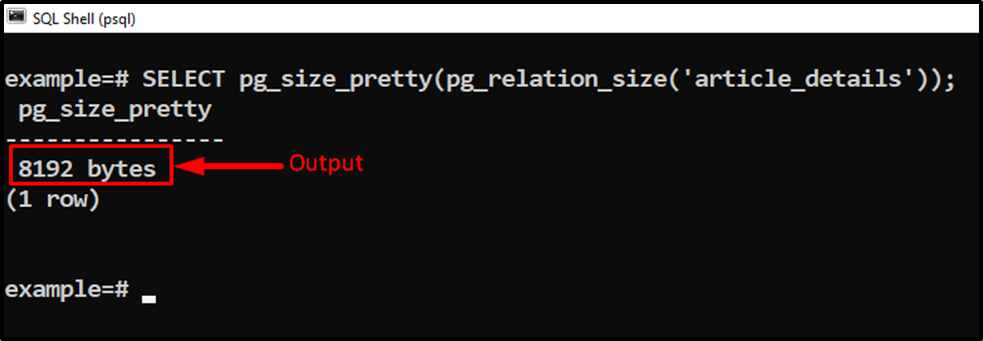This way, users can get the table’s size in a more clear way.

Step 5: Get Total Size

Use the pg_total_relation_size instead of pg_relation_size to get total size of the table including indexes and some other additional objects:

`SELECT pg_size_pretty (pg_total_relation_size ('article_details'));`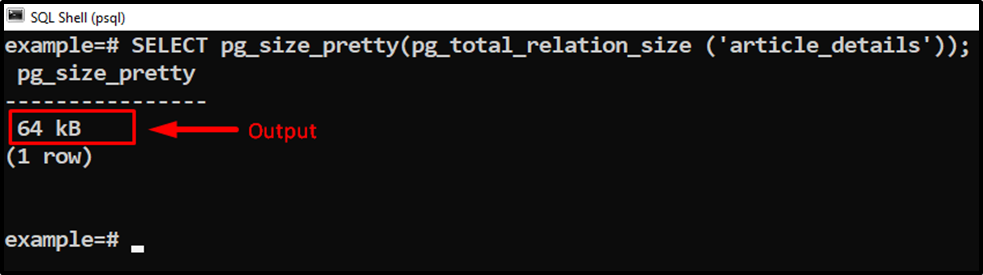This clearly shows that the pg_total_relation_size() retrieves the total table size, including indexes and other additional objects.

Conclusion

PostgreSQL provides a pg_relation_size() function to get the size of a particular table. Postgres offers another convenient function named “pg_size_pretty()” to get the table’s size in a human-readable format, such as bytes, KBs, MBs, and so on. The pg_relation_size() function retrieves only the table’s size without any additional object. However, to get the total size of a table, including indexes and some other additional objects, the pg_total_relation_size() is used in Postgres. This blog post demonstrated various functions that assist the users in getting the table size in Postgres.